﻿ 城市的力量：农民工层级迁移与工资溢价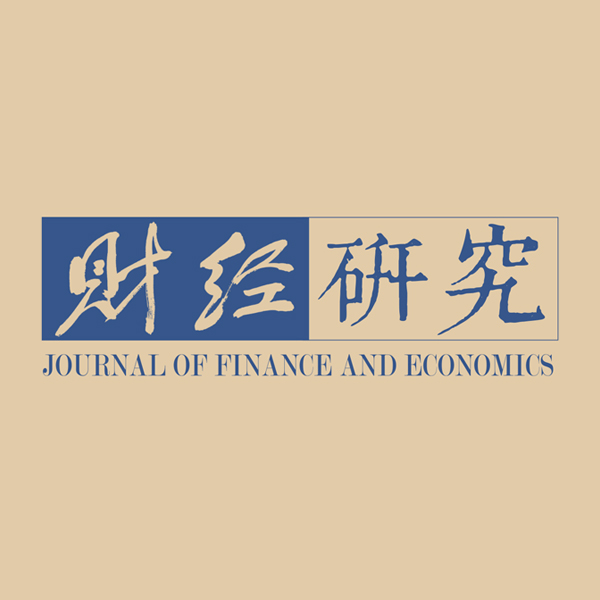《财经研究》
2021第47卷第2期

1. 上海财经大学 财经研究所，上海 200433;
2. 上海财经大学 三农研究院，上海 200433

The Power of Cities: Hierarchical Migration and Wage Premium of Migrant Workers
Hu Wen1,2, Zhang Jinhua1,21. Institute of Finance and Economics，Shanghai University of Finance and Economics，Shanghai 200433，China;
2. Research Institute of Agricultural，Farmer and Rural Society，Shanghai University of Finance and Economics，Shanghai 200433，China
Key words: administrative hierarchy of cities    hierarchical migration    wage premium    mechanism    quantile regression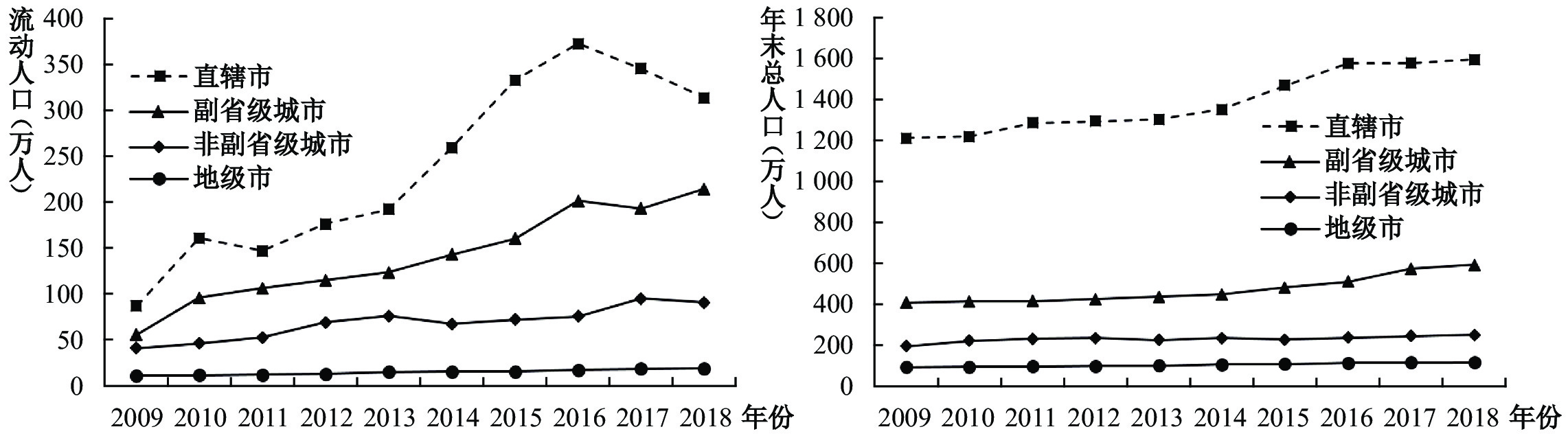图 1 2009−2018年中国不同行政级别城市的流动人口和年末总人口变动趋势 注：资料来源为历年《中国城乡建设统计年鉴》和《中国区域经济统计年鉴》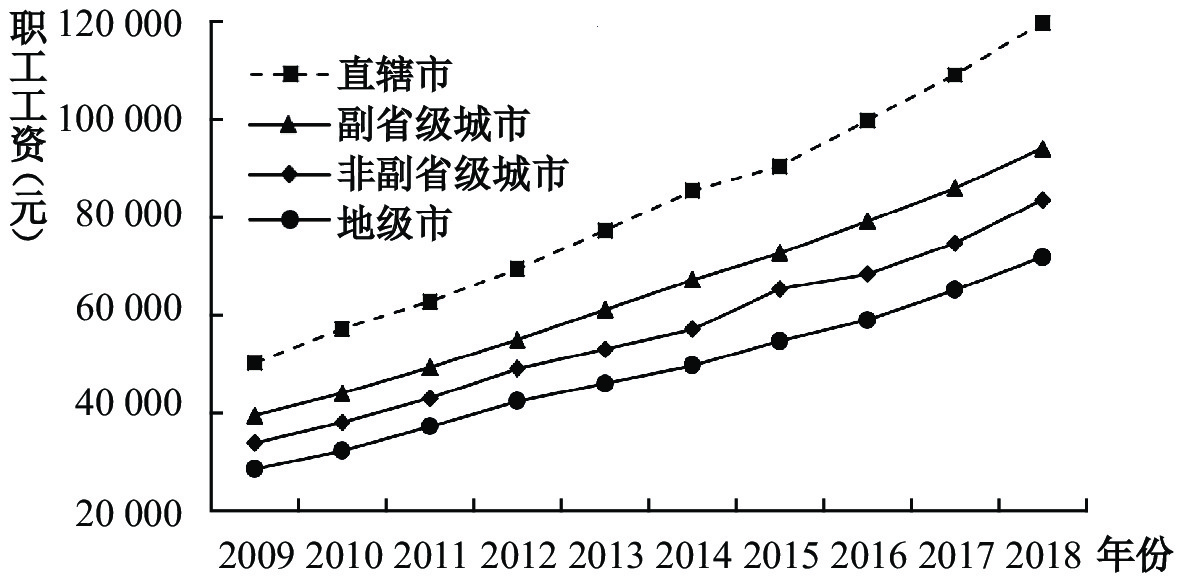图 2 2009−2018年中国不同行政级别城市的工资趋势

1. 消费者偏好。假设农民工在城乡劳动力市场中自由流动，农业部门和非农部门处于完全竞争经济体中，劳动力价格或要素价格在城市间相同，可标准化为1。在劳动力无弹性供给1单位时，城市劳动力供给量等于劳动力人口总数。那么，农民工的迁移决策函数为：

 \begin{aligned} & \max \;\; U = {x^\alpha }{y^\beta } \\ & {\rm{s.t.}} \;\;\; y + Px \leqslant W \\ \end{aligned} (1)

 \begin{aligned} & \dfrac{{\ln U - \ln C}}{{\alpha + \beta }} = \ln W - \theta \ln P \\ & C = \dfrac{{{\alpha ^\alpha }{\beta ^\beta }}}{{{{(\alpha + \beta )}^{\alpha + \beta }}}} \\ & \theta = \dfrac{\alpha }{{\alpha + \beta }} \end{aligned} (2)

$C$

$\theta$

 $u = w - \theta p$ (3)

 ${u_{ij}} = {w_{ij}} - \theta {p_j} + {e_{ij}}$ (4)

${e_{iL}} - {e_{iH}} \sim U[ - \mu ,\mu ]$
μ越大，意味着农民工的个人偏好对迁移地的选择就越重要。在均衡时，
${U_{iL}} - {U_{iH}}$
，可得
${e_{iL}} - {e_{iH}} = ({w_{iH}} - \theta {p_H}) - ({w_{iL}} - \theta {p_L})$
，进而得到两类城市的人口比例是：

 \begin{aligned} & \dfrac{{{n_L}}}{n} = \dfrac{{\mu - [({w_{iH}} - \theta {p_H}) - ({w_{iL}} - \theta {p_L})]}}{{2\mu }} \\ & \dfrac{{{n_H}}}{n} = \dfrac{{\mu + [({w_{iH}} - \theta {p_H}) - ({w_{iL}} - \theta {p_L})]}}{{2\mu }} \\ \end{aligned} (5)

${n_s}$

$s = H,L$
），
$n = {n_L} + {n_H}$

 ${w_{iH}} = {w_{iL}} + (\theta {p_H} - \theta {p_L}) + \mu \frac{{{n_H} - {n_L}}}{n}$ (6)

2. 住房市场。假设每个农民工消费1单位的住房，整个城市的住房需求为：

 \begin{aligned} {p_H} = & \dfrac{1}{\theta }({w_H} - {w_L}) + {p_L} - \frac{\mu }{\theta }\dfrac{{{n_H} - {n_L}}}{n} \\ {p_s} = & {\rho _s}{n_s},\;s = H,L,\;{\rho _s} = {\rho _H},{\rho _L} \\ \end{aligned} (7)

$\;{\rho _H} , {\rho _L}$

${\rho _s}$

3. 均衡。由式（6）、（7）可得模型的如下均衡解：

 \begin{aligned} {\lambda _s} = & n\theta {\rho _s}(1 - \beta ) + n(\alpha + \beta - 1) + \mu (1 - \beta ) \\ {w_s} = & - \frac{{1 - \alpha - \beta }}{{1 - \beta }}\left(1 - \frac{{{\lambda _s}}}{{{\lambda _H} + {\lambda _L}}}\right)n + C \\ {p_s} = & {\rho _s}\left(1 - \frac{{{\lambda _s}}}{{{\lambda _H} + {\lambda _L}}}\right){n_s} \\ \end{aligned} (8)

4. 比较静态分析。结合比较静态分析，对住房供给弹性求导，得到房价边际变化为：

 \begin{aligned} & \frac{{\partial {p_H}}}{{\partial {\rho _H}}} = \frac{{{\lambda _L}}}{{{{({\lambda _H} + {\lambda _L})}^2}}}n[{\lambda _L} + n(1 - \alpha - \beta ) + \mu (1 - \beta )] > 0 \\ & \frac{{\partial {p_L}}}{{\partial {\rho _H}}} = \frac{{{\lambda _L}}}{{{{({\lambda _H} + {\lambda _L})}^2}}}{\rho _L}{n^2}\theta (1 - \beta ) > 0 \\ & \frac{{\partial {p_H}}}{{\partial {\rho _H}}} - \frac{{\partial {p_L}}}{{\partial {\rho _H}}} = \frac{{{\lambda _L}}}{{{{({\lambda _H} + {\lambda _L})}^2}}}[2n(1 - \alpha - \beta ) + 2\mu (1 - \beta )] > 0 \\ \end{aligned} (9)

 \begin{aligned} & \dfrac{{\partial {w_H}}}{{\partial {\rho _H}}} = \dfrac{{1 - \alpha - \beta }}{{1 - \beta }}\dfrac{{{\lambda _L}}}{{{{({\lambda _H} + {\lambda _L})}^2}}}{n^2}\theta (1 - \beta ) > 0 \\ & \dfrac{{\partial {w_L}}}{{\partial {\rho _H}}} = \dfrac{{1 - \alpha - \beta }}{{1 - \beta }}\dfrac{{{\lambda _L}}}{{{{({\lambda _H} + {\lambda _L})}^2}}}{n^2}\theta (1 - \beta ) < 0 \end{aligned} (10)

（一）模型与策略

1. OLS回归。在基准的Mincer（1958）工资决定方程中，引入“城市行政层级”这一关键解释变量，采用普通最小二乘法估计如下的线性回归模型：

 \begin{aligned} & Ran{k_{ij}} = \alpha + \gamma {X_i} + {\varepsilon _i} \\ & Wag{e_{ij}} = \alpha + {\beta _{\rm{1}}}Ran{k_{ij}} + \gamma {X_i} + \tau {Z_j} + {\varepsilon _i} \\ \end{aligned} (11)

2. 分位数回归。采用分位数回归，能在0.10、0.25、0.50、0.75和0.90分位数上，分析迁移层级的工资溢价效应。方程如下：

 $Q_{q}(Y \mid X)=X_{i}^{\prime} \beta_{q}$ (12)

$Q_{q}(Y \mid X)$

3. 机制研究。根据中介效应法（Sobel，1982；温忠麟等，2004），进行作用机制检验：

 $Wag{e_{ij}} = \alpha + {\beta _{\rm{1}}}Ran{k_{ij}} + {\beta _{\rm{1}}}Cit{y_{ij}} + \gamma {X_i} + \tau {Z_j} + {\varepsilon _i}$ (13)
 $C i t y_{i j}=\alpha+\beta_{1} Z_{j}+\varepsilon_{i j}$ (14)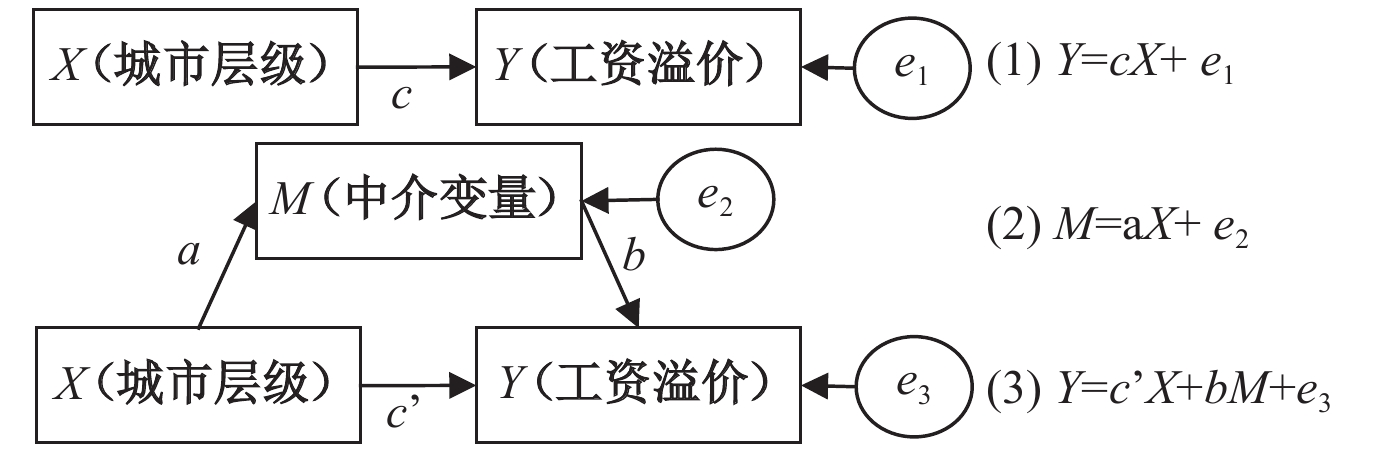图 3 中介变量的作用机制图

（二）数据与变量

 变量 2013年 2019年 观测值 % 月工资 小时工资 观测值 % 月工资 小时工资 直辖市 508 11.39 3111.89 16.14 555 14.23 5967.72 27.08 副省级城市 1467 32.89 2726.24 14.48 1085 27.82 4094.34 18.85 省会城市 434 9.73 3719.24 21.22 503 12.90 4449.14 20.44 普通地级市 2051 45.99 2670.39 13.79 1757 45.05 4077.21 18.87

 变量 定义 2013年 2019年 均值 标准差 均值 标准差 月工资 Ln（月均工资）（元） 7.74 0.56 8.12 0.76 小时工资 Ln（小时工资）（元） 2.35 0.69 2.76 0.74 行政层级 4=直辖市；3=副省级城市；2=省会城市；1=普通地级市 2.10 1.11 2.11 1.13 城市等级 5=一线；4=二线；3=三线；2=四线；1=五线 3.27 1.39 3.93 1.30 城市规模 5=超大城市；4=特大城市；3=大城市；2=中城市；1=小城市 3.39 1.17 3.68 1.33 人口规模 Ln（城市的人口规模）（万人） 5.61 0.90 5.84 1.26 文化程度 正规受教育的年限 8.20 3.30 3.03 1.00 健康状况 由1至4表示健康状况差到好 3.22 0.78 4.15 0.90 性别 男=1，女=0 0.68 0.47 0.85 0.36 工作经验 务工的时间年限 5.24 5.73 27.82 8.98 流动频率 工作转换第几个城市 0.37 0.87 1.94 2.32 社会资本 求职途径：亲友帮忙=1，自己求职=0 0.46 0.50 0.22 0.41 就业性质 国有企业=1，私有企业=0 0.10 0.30 0.14 0.34 住房价格 Ln（住宅商品房销售额/销售面积）（元/平方米） 8.97 0.63 9.17 0.72 基础设施 Ln（人均城市道路面积）（平方米） 2.64 0.61 2.73 0.45 教育设施 Ln（市辖区高等学校专任教师数）（人） 8.97 1.52 10.37 1.03 医疗设施 Ln（市辖区医院数量）（个） 5.36 2.25 4.77 1.01 注：数据来源为上海财经大学2013年和2019年千村调查，2012年和2018年《中国区域经济统计年鉴》《中国城市统计年鉴》。城市规模分类标准依据《国务院关于调整城市规模化分标准的通知》（国发51号）文件所示，以城区常住人口为统计口径，数据来源于《中国城市建设统计年鉴》中城区人口+城区暂住人口计算常住人口规模，按照规模大小分为5=超大城市（1000万以上）；4=特大城市（500—1000万）；3=大城市（100—500万）；2=中等城（50—100万）；1=小城市（50万以下）。

1. 被解释变量为农民工小时工资（对数）。农民工的月均工资和小时工资存在度量误差，不同行业、职业的每周工作小时不同，农民工的工作小时数普遍高于城市居民，所以月均工资和小时工资与城市居民有较大差异，小时工资更能反映劳动力价格的差异。由表1可知，2013年的省会城市样本的月工资均值最高，直辖市次之，普通地级市的工资最低；2019年的直辖市和省会城市的工资要高于普通地级市的工资水平。

2. 核心解释变量为城市行政层级。本文将“普通地级市”定义为1，“非副省级省会”定义为2，“副省级城市”定义为3，直辖市定义为4。2013年4类迁移层级农民工样本分别为2051、434、1467和508，2019年分别为1757、503、1085和555。此外，本文按照城市规模、城市等级划分的数量和比例来看，迁移到直辖市、副省级城市、省会城市高于普通地级市，迁移到中小城市和四五线城市的农民工远远少于迁移到大城市、高级别城市的农民工数量。

3. 个体特征变量。包括受教育年限、健康状况、性别、就业性质、工作经验、社会资本和流动频率。就业性质从就业单位所属性质为国有还是私有来判定，其中国有企业包括“国有及控股企业”，私有企业包括“私营企业”“中外合资及外商独资企业”。

4. 城市特征变量。城市交通设施用人均道路铺装面积度量，教育资源用市辖区的高等院校专任教师衡量，医疗设施用医院数量来反映一个城市的公共服务状况。

5. 工具变量。本文选用“1984年人口规模”作为历史工具变量，估计城市行政级别对工资的弹性，缓解内生性问题。原因在于：一是1984年相对于2012年的城市数据而言，滞后将近30年的人口规模反映了现在经济活动集聚的基础，也能作为历史信息的变量。二是在数据可得性方面，1985年《中国城市统计年鉴》是能追溯到较早、较全面的年鉴。三是1984年开始形成中国的城市级别架构，划分为直辖市、副省级城市、非副省级省会城市和普通地级市。1984年10月20日，党的十二届三中全会通过了《中共中央关于经济体制改革的决定》，提出“加快以城市为重点的整个经济体制改革的步伐”，强调“要充分发挥城市的中心作用，逐步形成以城市特别是大、中城市为依托的，不同规模、开放式、网络型的经济区”，标志着城市经济体制改革的大幕全面拉开。所以1984年这一节点的人口规模对中国城市格局具有重要影响（江艇等，2018），又不会直接影响现在的工资水平，所以该变量也是外生的。因此，本文采用“1984年人口规模”作为历史滞后工具变量进行检验。

（一）基准回归结果：层级迁移

 2013年 2019年 （1）行政层级 （2）城市等级 （3）城市规模 （4）人口规模 （5）行政层级 （6）城市等级 （7）城市规模 （8）人口规模 子女随迁 −0.213*** −0.323*** −0.283*** −0.157*** −0.313*** −0.444*** −0.411*** −0.382*** （0.048） （0.059） （0.049） （0.039） （0.101） （0.115） （0.118） （0.111） 配偶随迁 0.052 0.239*** 0.106** 0.047 0.178*** 0.416*** 0.291*** 0.240*** （0.043） （0.053） （0.044） （0.035） （0.064） （0.073） （0.075） （0.071） 年龄 −0.000 −0.010*** −0.009*** −0.004** 0.012 −0.006 −0.003 0.003 （0.002） （0.003） （0.002） （0.002） （0.016） （0.018） （0.018） （0.017） 婚姻 −0.095* −0.184*** −0.171*** −0.078* −0.017 0.049 0.042 0.016 （0.053） （0.066） （0.055） （0.043） （0.058） （0.066） （0.068） （0.064） 文化程度 0.013** 0.004 0.002 0.014*** 0.089*** 0.117*** 0.138*** 0.139*** （0.005） （0.007） （0.006） （0.004） （0.019） （0.022） （0.023） （0.022） 家庭规模 0.007 0.043*** 0.057*** 0.015* 0.088 0.220*** 0.128* 0.131* （0.009） （0.011） （0.010） （0.007） （0.063） （0.071） （0.073） （0.070） 工作经验 0.007** 0.016*** 0.013*** 0.007*** −0.016 −0.004 −0.005 −0.010 （0.003） （0.004） （0.003） （0.003） （0.015） （0.018） （0.018） （0.017） 流动频率 −0.022 −0.018 −0.078*** −0.032** −0.020** −0.015* −0.026*** −0.033*** （0.019） （0.024） （0.020） （0.016） （0.008） （0.009） （0.009） （0.009） Constant 2.024*** 3.347*** 3.459*** 5.588*** 1.753*** 3.766*** 3.457*** 5.507*** （0.102） （0.126） （0.105） （0.083） （0.281） （0.320） （0.331） （0.313） N 4460 4460 4460 4460 3900 3900 3853 3853 R2 0.009 0.027 0.038 0.016 0.014 0.029 0.025 0.026 注：***、**和*分别为系数在1%、5%和10%的显著性水平显著；括号内为稳健标准误。

（二）基准回归结果：工资溢价

 2013年 2019年 （1）月均工资 （2）月均工资 （3）小时工资 （4）小时工资 （5）月均工资 （6）月均工资 （7）小时工资 （8）小时工资 行政层级 0.041*** −0.036** 0.060*** −0.037** 0.053*** 0.142*** 0.048*** 0.110*** （0.007） （0.014） （0.009） （0.017） （0.010） （0.027） （0.010） （0.027） 住房价格 0.113*** 0.111*** 0.041* 0.042* （0.021） （0.025） （0.024） （0.024） 人均道路 −0.025* −0.049*** 0.008*** 0.006*** （0.014） （0.018） （0.002） （0.002） 教育设施 0.053*** 0.074*** −0.000*** −0.000*** （0.010） （0.012） （0.000） （0.000） 医疗设施 −0.024*** −0.026*** 0.058** 0.043* （0.005） （0.006） （0.025） （0.025） Constant 6.957*** 5.842*** 1.432*** 0.257 7.136*** 6.384*** 1.828*** 1.197*** （0.048） （0.181） （0.058） （0.217） （0.115） （0.258） （0.113） （0.256） 个体特征 控制 控制 控制 控制 控制 控制 控制 控制 N 4460 4460 4460 4460 3900 3544 3900 3544 R2 0.103 0.115 0.088 0.099 0.095 0.110 0.087 0.094 注：***、**和*分别为系数在1%、5%和10%的显著性水平显著；括号内为稳健标准误。

 （1）OLS （2）QR_10 （3）QR_25 （4）QR_50 （5）QR_75 （6）QR_90 2013年：只控制个体特征 0.026***（0.004） 0.035***（0.006） 0.031***（0.004） 0.029***（0.004） 0.024***（0.004） 0.016**（0.008） 2013年：控制个体和城市特征 0.012*（0.007） 0.012（0.010） 0.013*（0.008） 0.015**（0.007） 0.016**（0.008） -0.004（0.014） 2019年：只控制个体特征 0.053***（0.010） 0.029（0.027） 0.025*（0.015） 0.040***（0.009） 0.058***（0.010） 0.064***（0.014） 2019年：控制个体和城市特征 0.142***（0.027） 0.195***（0.068） 0.071*（0.038） 0.072***（0.024） 0.097***（0.027） 0.114***（0.034） 注：***、**和*分别为系数在1%、5%和10%的显著性水平显著；括号内为稳健标准误。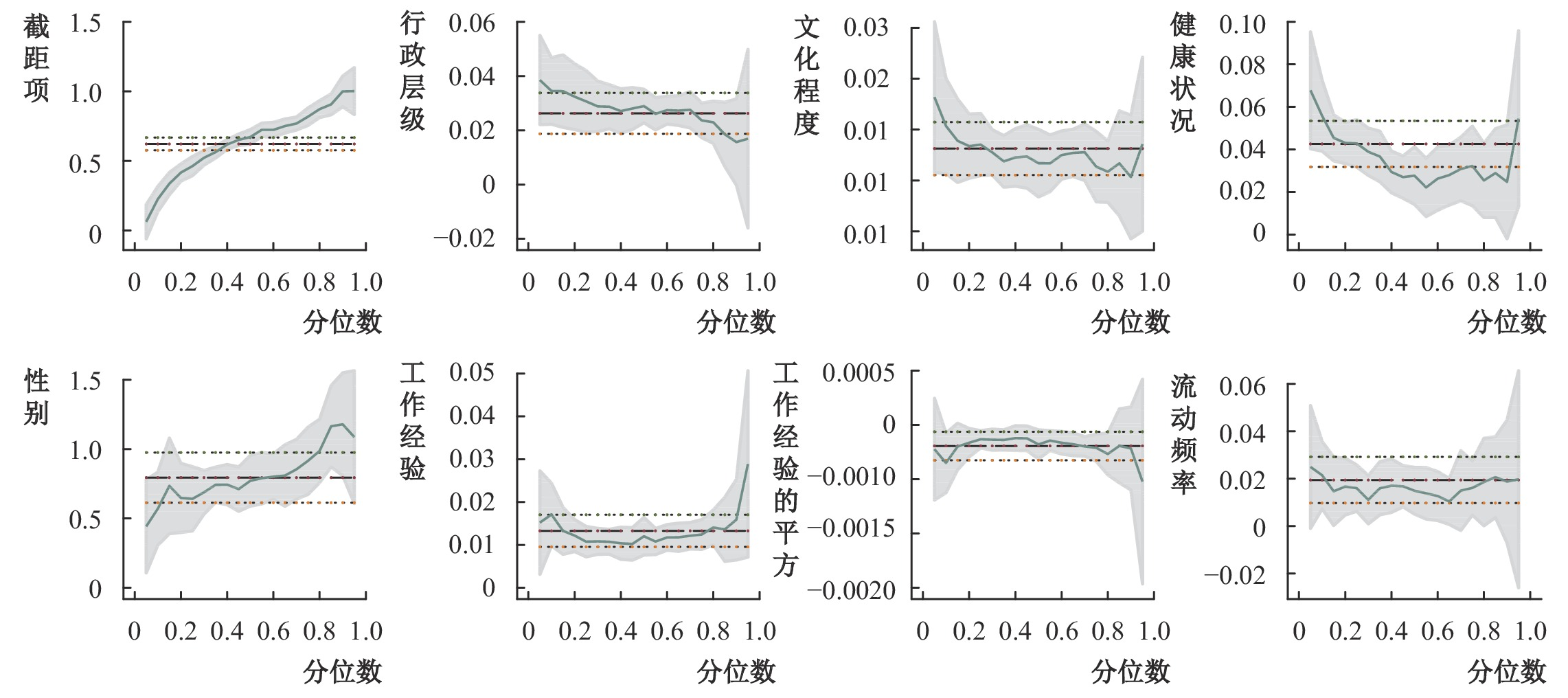图 4 农民工工资分位数回归结果（2013年）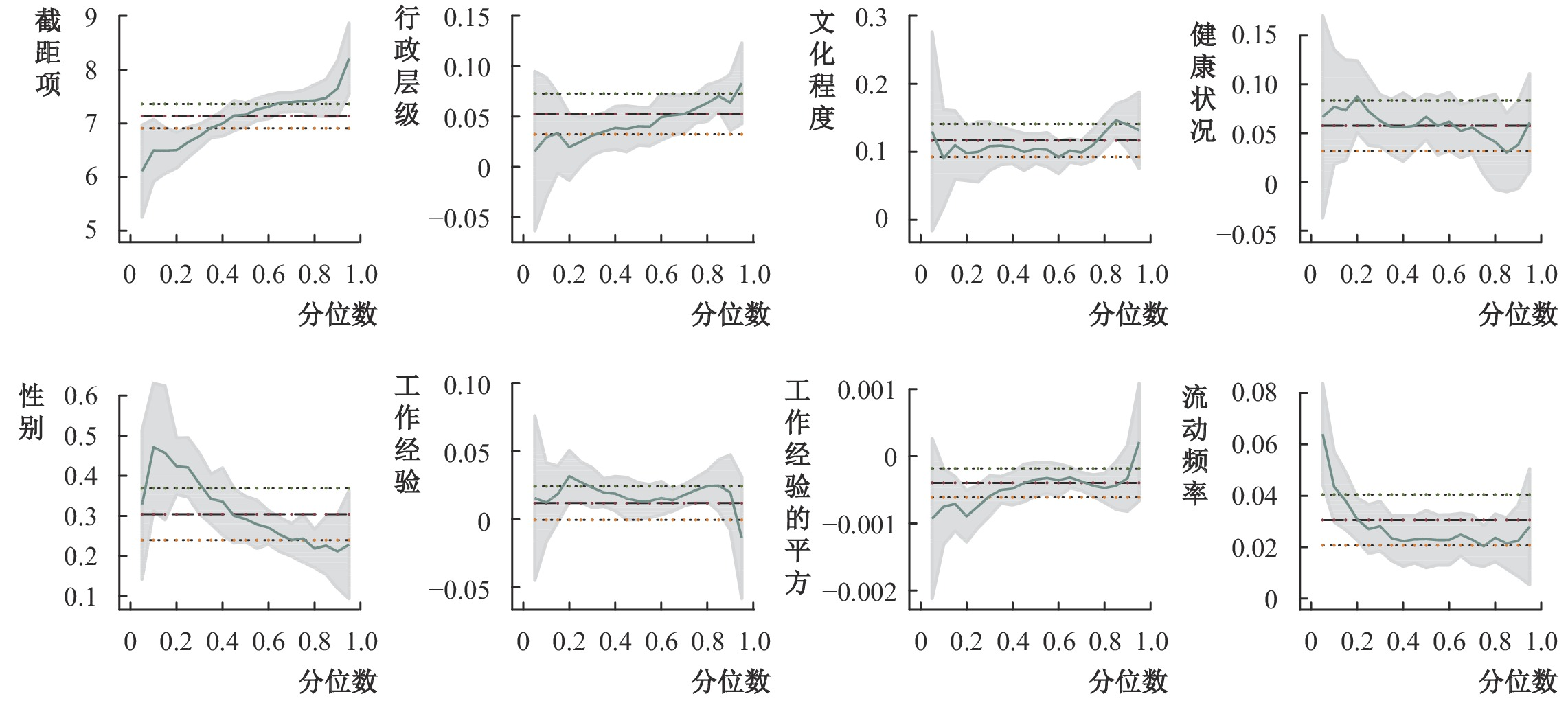图 5 农民工工资分位数回归结果（2019年）

（三）内生性检验：行政层级的工具变量

 月均工资 小时工资 （1） （2） （3） （4） 一阶段回归结果First-Stage 1984年城市规模 0.766***（0.014） 0.178***（0.010） 0.766***（0.014） 0.178***（0.010） 二阶段回归结果2SLS 城市行政级别 0.085***（0.015） 0.678***（0.116） 0.086***（0.015） 0.664***（0.111） 个体特征 控制 控制 控制 控制 城市特征 控制 控制 N 3517 3353 3517 3353 R2 0.094 0.010 0.085 0.860 DWH检验 chi2（1）=17.59（p = 0.000） chiDWH检验26.22（p = 0.000） chi2（1）=19.30（p= 0.000） chi2（1）=29.04（p = 0.000） KRF检验 F= 3193.18（p = 0.000） F= 294.35（p = 0.000） F= 3193.18（p= 0.000） F= 294.35（p = 0.000） 注：（1）***、**和*分别为系数在1%、5%和10%的显著性水平显著；括号内为稳健标准误。（2）数据来源：作者计算整理。

（四）中介效应检验：城市层级的行政力量VS市场力量

 2013年 2019年 （1） （2） （3） （4） （5） （6） （7） （8） 城市行政层级 0.014**（0.006） 0.026***（0.004） 0.005 （0.006） 0.030***（0.005） 0.065***（0.014） 0.062***（0.013） 0.121***（0.022） 0.032 （0.020） 住房价格 0.030***（0.010） −0.043*（0.022） 人均道路 −0.002 （0.007） 0.028 （0.033） 教育设施 0.020***（0.005） −2.38e-06***（6.03e-07） 医疗设施 −0.003 （0.002） 0.020 （0.020） 个体特征 控制 控制 控制 控制 控制 控制 控制 控制 N 4460 4460 4460 4460 3849 3853 3593 3548 R2 0.089 0.088 0.093 0.089 0.074 0.073 0.074 0.070 F-test 44.04*** 43.11*** 45.33*** 43.29*** 33.86*** 33.79*** 31.93*** 29.71*** Sobel检验 （Z值） 2.428*** −1.784*** 0.737 6.313*** 4.700*** −4.615*** 5.509*** 1.596*** Sobel检验 （系数） 0.018 −0.001 0.003 0.008 0.069 −0.098 2.921e-06 0.031 中介效应/总效应 38.15% 36.47% 11.33% 157.89% 157.95% 136.49% 543.11% 60.98% 注：***、**和*分别为系数在1%、5%和10%的显著性水平显著；括号内为稳健标准误；Sobel检验的Z值大于0.97或小于-0.97，说明中介效应显著。

（五）异质性考察

 2013年 2019年 （1）新生代 （2）老一代 （3）男性 （4）女性 （5）新生代 （6）老一代 （7）男性 （8）女性 行政层级 0.026***（0.004） 0.026***（0.009） 0.027***（0.005） 0.025***（0.006） 0.037**（0.015） 0.057***（0.014） 0.051***（0.011） 0.029 （0.027） Constant 0.652***（0.027） 0.578***（0.049） 0.673***（0.028） 0.687***（0.039） 1.516***（0.195） 2.141***（0.449） 2.020***（0.124） 2.003***（0.278） N 3363 1097 3023 1437 1487 2413 3318 582 R2 0.093 0.092 0.073 0.069 0.085 0.077 0.069 0.125 F-test 38.04*** 12.18*** 29.52*** 13.24*** 15.24*** 22.23*** 30.74*** 10.18*** 注：***、**和*分别为系数在1%、5%和10%的显著性水平显著；括号内为稳健标准误。

① 十八大报告中首次提出“优化行政层级和行政区划设置，有条件的地方可探索省直接管理县（市）改革”，是中央层面改革行政管理体制的重要信号。国家发展和改革委员会发布《2019年新型城镇化建设重点任务》，提出继续加大户籍制度改革力度，对各类人口规模城市的落户政策均进行提档升级。

② 数据来源于1985年《中国城市统计年鉴》，剔除33个因行政区划变动缺失数据的城市：巴彦淖尔、巴中、白银、亳州、定西、东莞、鄂尔多斯、防城港、贵港、葫芦岛、淮安、揭阳、晋中、荆州、酒泉、来宾、丽水、陇南、吕梁、宁德、攀枝花、盘锦、清远、日照、商洛、松原、台州、乌兰察布、武威、宿迁、宣城、榆林、资阳。

③ 1984年7月18日，国家计划委员会发布《关于重庆市、武汉市、沈阳市、大连市计划单列的通知》。

④ 王振川主编，中国共产党十二届三中全会通过《中共中央关于经济体制改革的决定》[Z].中国改革开放新时期年鉴，1984年。

  爱德华·格莱泽. 城市的胜利[M]. 刘润泉译. 上海: 上海社会科学院出版社, 2012.  蔡昉, 都阳. 转型中的中国城市发展——城市级层结构、融资能力与迁移政策[J]. 经济研究, 2003(6): 64–71，95.  黄枫, 吴纯杰. 中国省会城市工资溢价研究——基于分位数回归的空间计量分析[J]. 财经研究, 2008(9): 71–80. DOI:10.3969/j.issn.1001-9952.2008.09.007  黄燕芬, 张超. 城市行政层级视角的人口流动影响机理研究[J]. 中国人口科学, 2018(1): 33–45.  江艇, 孙鲲鹏, 聂辉华. 城市级别、全要素生产率和资源错配[J]. 管理世界, 2018(3): 38–50，77. DOI:10.3969/j.issn.1002-5502.2018.03.004  温忠麟, 张雷, 侯杰泰, 等. 中介效应检验程序及其应用[J]. 心理学报, 2004(5): 614–620.  踪家峰, 周亮. 大城市支付了更高的工资吗?[J]. 经济学（季刊）, 2015(4): 1467–1496.  Duranton G, Puga D. Micro-foundations of urban agglomeration economies[J]. Handbook of Regional and Urban Economics, 2004, 4: 2063–2117. DOI:10.1016/S1574-0080(04)80005-1  Glaeser E L, Maré D C. Cities and skills[J]. Journal of Labor Economics, 2001, 19(2): 316–342. DOI:10.1086/319563  Mincer J. Investment in human capital and personal income distribution[J]. Journal of Political Economy, 1958, 66(4): 281–302. DOI:10.1086/258055  Moretti E. Local labor markets[R]. IZA Discussion Papers, No.4905, 2010.  Roback J. Wages, rents, and the quality of life[J]. Journal of Political Economy, 1982, 90(6): 1257–1278. DOI:10.1086/261120  Rosen S. Wage-based indexes of urban quality of life[C]. Mieszkowski P, Strazheim M. Current issues in urban economics[M]. Baltimore: Johns Hopkins University Press, 1979.  Sobel M E. Asymptotic confidence intervals for indirect effects in structural equation models[J]. Sociological Methodology, 1982, 13: 290–312. DOI:10.2307/270723  Xing B C, Zhang J F. The preference for larger cities in China: Evidence from rural-urban migrants[J]. China Economic Review, 2017, 43: 72–90. DOI:10.1016/j.chieco.2017.01.005